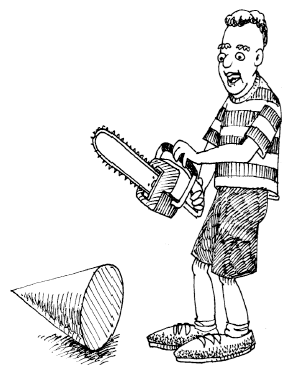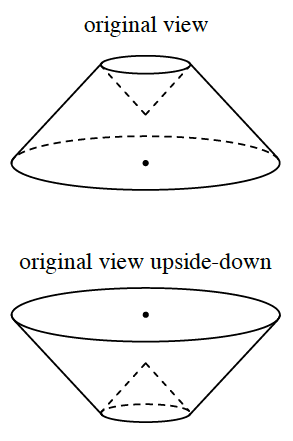### Home > CCG > Chapter 11 > Lesson 11.1.5 > Problem11-75

11-75.Martha was playing with a hollow plastic cone that had a diameter of $12$ inches and a height of $5$ inches. Her brother Matt snatched the cone, cut two inches off the top, and placed the cut piece upside down in the lower portion of the cone as shown at right. How many cubic inches of water would fit inside the space of her redesigned cone?$\array{ \qquad \qquad \quad \text{Volume of the original cone} \\ \quad \ - \quad \text{Volume of smaller cone}\\ \hline = \ \ \ \text{Volume of new cone} }$

${\large{V}}\text{cone} = \frac{1}{3} \pi r^2h$

Use a proportion ratio to solve for the radius of the smaller cone.

$\approx164.37\text{ in}^3$